• 压缩感知重构Matlab重构算法MATLAB编写、含有SP算法、各类重构算法
• 数据信号处理matlab，程序实现压缩感知重构过程，仅供参考。
• 最近在看压缩感知重构算法，试了其中一些，把代码链接给大家列一下： TwIST: http://www.lx.it.pt/~bioucas/code.htm GPSR: http://www.lx.it.pt/~mtf/GPSR/ OMP: http://www.eee.hku.hk/~wsha/Freecode/Files...
展开全文压缩感知
• 二维图像（二维图像的压缩感知重构算法程序代码、内含完整的MATLAB代码）
• 6压缩感知（Compressed sensing），也被称为压缩采样（Compressive sampling）或稀疏采样（Sparse sampling），是一种寻找欠定线性系统的稀疏解的技术。
• matlab平台编写的基于压缩感知的信道估计重构算法的实现
• 利用MATLAB实现了压缩感知重构算法，里面包含相应的函数及解释，对学习该类算法有一个较好的认识，有利于学习
• 压缩感知重构信号,压缩感知重构算法,matlab源码.rar
• 压缩感知重构信号,压缩感知重构算法,matlab源码.zip
• 压缩感知OMP重构算法matlab实现，OMP重构算法，本程序用于重构原始图像
• 压缩感知之OMP恢复算法 1、基本思想 　y=Φx x=Ψθ 　正交匹配追踪算法的本质思想是，以贪婪迭代的方式选择测量矩阵Φ的列，使得在每次迭代中所选择的列与当前的冗余向量最大程度地相关，从测量向量中减去相关...

压缩感知之OMP恢复算法

1、基本思想

y=Φx 　　x=Ψθ
正交匹配追踪算法的本质思想是，以贪婪迭代的方式选择测量矩阵Φ的列，使得在每次迭代中所选择的列与当前的冗余向量最大程度地相关，从测量向量中减去相关部分并反复迭代，直到迭代次数达到稀疏度K，强制迭代停止。

2、算法步骤

输入：（1）M*N的感知矩阵A，其中M远远小于N，A=Φ*Ψ。
（2）长度为M的数据向量b，即测量值y。
输出：长度为N的重建向量 xˆ $\widehat {\rm{x}}$，满足y=Ax。
初始化：残差r0=y，重建信号x0=0，索引集Λ0=Φ，迭代次数n=2*K，计数器k=0。
步骤1：计算残差和感知矩阵A的每一列的投影系数（内积值） ck=ATrk1 ${{\rm{c}}_{\rm{k}}}{\rm{ = }}{{\rm{A}}^T}{{\rm{r}}_{{\rm{k - 1}}}}$
步骤2：找出ck中元素最大的元素 ck=max{ck} ${\rm{c}}_{\rm{k}}^{\rm{*}}{\rm{ = }}\max \left\{ {{{\rm{c}}_{\rm{k}}}} \right\}$以及对应的位置pos；
步骤3：更新索引集 Λk=Λk1{pos}, ${\Lambda _{\rm{k}}}{\rm{ = }}{\Lambda _{{\rm{k - 1}}}} \cup \left\{ {{\rm{pos}}} \right\},$以及原子集合 AΛK=AΛk1{A(:pos)} ${A_{{\Lambda _{\rm{K}}}}}{\rm{ = }}{A_{{\Lambda _{{\rm{k - 1}}}}}} \cup \left\{ {A\left( {:{\rm{pos}}} \right)} \right\}$
步骤4：利用最小二乘求得近似解 xk=(ATΛkAΛk1)1ATΛky ${{\rm{x}}_{\rm{k}}}{\rm{ = }}{\left( {A_{{\Lambda _{\rm{k}}}}^TA_{{\Lambda _{{\rm{k - 1}}}}}^{}} \right)^{ - 1}}A_{{\Lambda _{\rm{k}}}}^T{\rm{y}}$
步骤5：更新余量 rk=yAxk ${{\rm{r}}_{\rm{k}}}{\rm{ = y - }}A{{\rm{x}}_k}$
步骤6：判断迭代是否满足停止条件，满足则停止 xˆ=xk,r=rk $\widehat x = {x_k},r = {r_k}$， 输出 xˆ,r $\widehat x,r$ ，否则转步骤1。

3、仿真验证

3.1 一维时间稀疏信号
首先进行一维时间稀疏信号的恢复，信号长度为512，稀疏度选取10、20、30、40、50，matlab代码如下：

clc;clear;close all
%%  1. 时域测试信号生成
CNT = 100;                                       %对于每组(K,M,N)，重复迭代次数
N=512;                                           %信号长度
K_set= [10,20,30,40,50];                             %信号x的稀疏度集合
Percentage = zeros(length(K_set),N);             %存储恢复成功概率
for kk=1:length(K_set)
K=K_set(kk);                                      %本次稀疏度
M_set=1:5:N;                                          %测量数每隔五个取一次
PercentageK = zeros(1,length(M_set));                 %存储此稀疏度K下不同M的恢复成功概率
for mm=1:length(M_set)
M=M_set(mm);                                      %本次观测次数
P=0;
for cnt=1:CNT                             %每个观测值个数均运行CNT次
Index_K=randperm(N);                              %将1-N随机打乱 行向量
x=zeros(N,1);
x(Index_K(1:K))=5*randn(K,1);                     %x为K稀疏的，且位置是随机的

%%  2.  时域信号压缩传感
Phi=randn(M,N);                                   %  测量矩阵(高斯分布白噪声)
Phi = Phi./repmat(sqrt(sum(Phi.^2,1)),[floor(M),1]); %正则化
y=Phi*x;                                        %  获得线性测量

%%  3.  正交匹配追踪法重构信号(本质上是L_1范数最优化问题)
n=2*K;                                            %  算法迭代次数(m>=K)
Psi=eye(N);                                       %  单位矩阵为正变换矩阵
A=Phi*Psi;                                        %  恢复矩阵(测量矩阵*正交反变换矩阵)

hat_y=zeros(1,N);                                 %  待重构的变换域里的向量
Aug_t=[];                                         %  增量矩阵(初始值为空矩阵)
r0=y;                                             %  残差值

for times=1:n;                                    %  迭代次数(有噪声的情况下,该迭代次数为K)
for col=1:N;                                  %  恢复矩阵的所有列向量    步骤1
product(col)=abs(A(:,col)'*r0);           %  恢复矩阵的列向量和残差的投影系数(内积值)
end
[val,pos]=max(product);                       %  最大投影系数对应的位置  步骤2
Aug_t=[Aug_t,A(:,pos)];                       %  矩阵扩充               步骤3 更新原子集合
A(:,pos)=zeros(M,1);                          %  选中的列置零（实质上应该去掉，为了简单我把它置零）
aug_y=(Aug_t'*Aug_t)^(-1)*Aug_t'*y;           %  最小二乘,使残差最小     步骤4 求近似解
r0=y-Aug_t*aug_y;                             %  残差                   步骤5 更新余量
pos_array(times)=pos;                         %  纪录最大投影系数的位置  步骤3 更新索引集
end
hat_y(pos_array)=aug_y;                           %  重构的变换域里的向量
hat_x=real(Psi'*hat_y.');                         %  做逆变换重构得到时域信号
if norm(hat_x-x)<1e-6                   %如果残差小于1e-6则认为恢复成功
P = P + 1;
end
end
PercentageK(mm) = P/CNT;                  %计算恢复概率
end
Percentage(kk,1:length(M_set)) = PercentageK;
end
save MtoPercentage100 %运行一次不容易，把变量全部存储下来
%}
%% 绘制概率图
S = ['-ks';'-ko';'-kd';'-kv';'-k*'];
figure;
for kk = 1:length(K_set)
K = K_set(kk);
M_set = 1:5:N;
L_Mset = length(M_set);
plot(M_set,Percentage(kk,1:L_Mset),S(kk,:));%绘出x的恢复信号
hold on;
end
hold off;
xlim([0 256]);
legend('K=10','K=20','K=30','K=40','K=50');
xlabel('Number of measurements(M)');
ylabel('Percentage recovered');
title('Percentage of input signals recovered correctly(N=256)(Gaussian)');

%%  4.  恢复信号和原始信号对比
% figure(1);
% hold on;
% plot(hat_x,'k.-')                                 %  重建信号
% plot(x,'r')                                       %  原始信号
% legend('Recovery','Original')
% norm(hat_x.'-x)/norm(x)                           %  重构误差

程序代码改变测量数得到恢复概率，如下图所示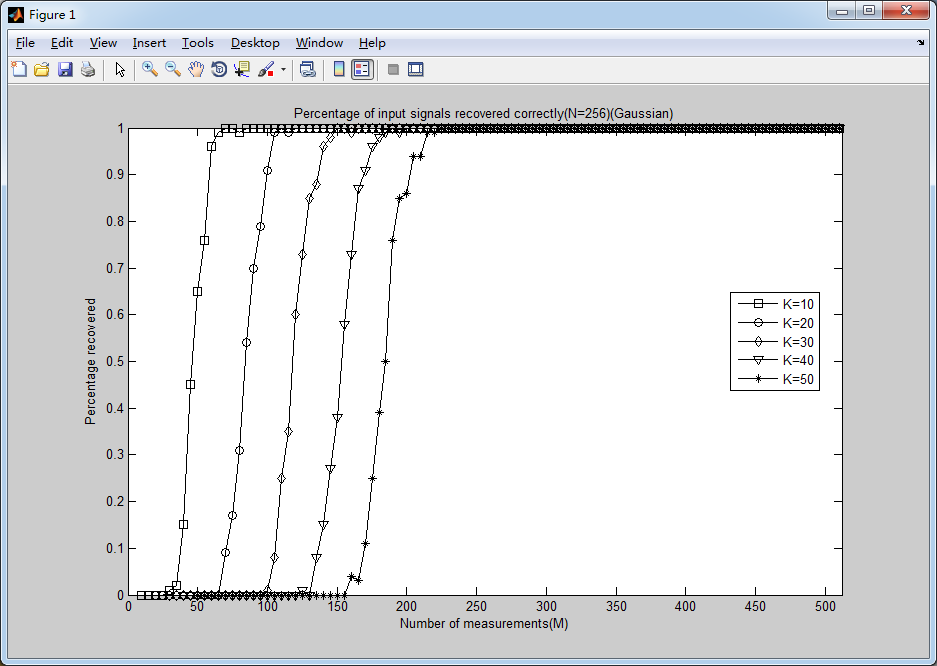《压缩感知及应用》书上的p68页图3-7如下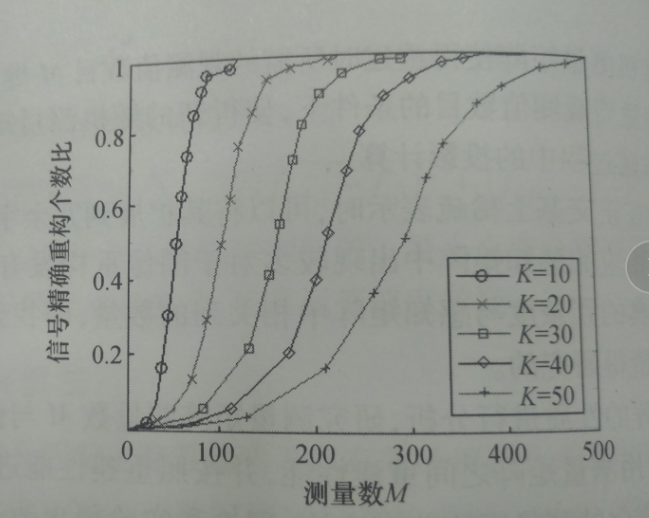结果要比《压缩感知及应用》书上p68页结果感觉略好，原因不详。
3.2 二维lena图像恢复
仿照《压缩感知及应用》这本书，p120页，进行lena图像恢复，压缩比使用0.3、0.4、0.5。所程序如下：

img=double(img);
[n,b]=size(img);             %文件为n行b列
figure(1)
subplot(2,2,1)
imshow(img,[])
weizhi=1;
Pm=zeros(3,1);           %放功率信噪比
Tm=zeros(3,1);           %放时间

for CR=0.3:0.1:0.5
disp(CR);
%  测量矩阵
m=floor(n*CR);
Phi=randn(m,n);                     %  测量矩阵生成
for i=1:n                           %  测量矩阵归一化
Phi(:,i) = Phi(:,i) / norm(Phi(:,i));%正则化测量矩阵φ
end

%  对图像进行欠采样
y=Phi*img;

%% CS重建（ 已知测量值y，测量矩阵Phi，稀疏基Psi'(小波变换矩阵) ）
Psi=DWT(n);                         %  小波变换矩阵生成(要求大小是2的整数幂次)%傅里叶正变换矩阵dftmtx(n)
A = Phi*Psi';                       %  y = Phi*X0 = Phi*Psi'*s（s是稀疏系数）此处X0=Psi's
y = y*Psi';                         %  此处不明白，为什么还要乘psi' 如果不乘，恢复效果很差

%OMP算法
tic
fprintf('OMP...\r\n');
ReS3 = zeros(n,b);
for i = 1:b
rec = omp(y(:,i),A,n);           %对y的的每一列进行重构，恢复变换域矩阵
ReS3(:,i) = rec;
end
T3 = toc;
ReS3 = Psi'*sparse(ReS3)*Psi;       %%%%%%%%%%%%%%%
ReImg3 = full(ReS3);

weizhi=weizhi+1;
subplot(2,2,weizhi)
imshow(ReImg3,[]);
%% 计算峰值噪声（PSNR）、用时

%   OMP
errorx=sum(sum(abs(ReImg3-img).^2));      %  MSE误差
psnr=10*log10(255*255/(errorx/n/b));%
Pm(weizhi-1,1)=psnr;
Tm(weizhi-1,1)=T3;

end
%% 显示结果

CR=0.3:0.1:0.5;
figure;
plot(CR,Pm(:,1),'ro');
hold on
plot(CR,Pm(:,1),'r');
xlabel('压缩比');
ylabel('峰值信噪比/dB');
axis([0.2,0.6,5,30]);

figure;
plot(CR,Tm(:,1),'g*')
hold on
plot(CR,Tm(:,1),'g')

xlabel('压缩比');
ylabel('运行时间/s');
axis([0.2,0.6,0,1+max(Tm(3,:))]);

function hat_y=omp(s,T,N)
%  OMP的函数
%  s-测量；T-观测矩阵；N-向量大小

Size=size(T);                                     %  观测矩阵大小
M=Size(1);                                        %  测量
hat_y=zeros(1,N);                                 %  待重构的谱域(变换域)向量
Aug_t=[];                                         %  增量矩阵(初始值为空矩阵)
r_n=s;                                            %  残差值

for times=1:M;                                    %  迭代次数（不会超过测量值）

for col=1:N;                                  %  恢复矩阵的所有列向量
product(col)=abs(T(:,col)'*r_n);          %  恢复矩阵的列向量和残差的投影系数(内积值)
end
[val,pos]=max(product);                       %  最大投影系数对应的位置
Aug_t=[Aug_t,T(:,pos)];                       %  矩阵扩充
T(:,pos)=zeros(M,1);                          %  选中的列置零（实质上应该去掉，为了简单我把它置零）
aug_y=(Aug_t'*Aug_t)^(-1)*Aug_t'*s;           %  最小二乘,使残差最小
r_n=s-Aug_t*aug_y;                            %  残差
pos_array(times)=pos;                         %  纪录最大投影系数的位置

if (abs(aug_y(end))^2/norm(aug_y)<0.0005)       %  自适应截断误差（***需要调整经验值）
break;
end
end

hat_y(pos_array)=aug_y;                           %  重构的向量

%  程序作者：沙威，香港大学电气电子工程学系，wsha@eee.hku.hk
%  参考文献：小波分析理论与MATLAB R2007实现，葛哲学，沙威，第20章  小波变换在矩阵方程求解中的应用（沙威、陈明生编写）.

%  构造正交小波变换矩阵，图像大小N*N，N=2^P，P是整数。

function ww=DWT(N)

[h,g]= wfilters('sym8','d');       %  获得symlets8小波的低通分解滤波器和高通分解滤波器的系数；
%  采用Symlets8的小波分解可得到较好的压缩比率及较高的信号恢复质量

% N=256;                           %  矩阵维数(大小为2的整数幂次)
L=length(h);                       %  滤波器长度
rank_max=log2(N);                  %  最大层数 8 ？
rank_min=double(int8(log2(L)))+1;  %  最小层数 5 ？
ww=1;   %  预处理矩阵

%  矩阵构造
for jj=rank_min:rank_max

nn=2^jj;

%  构造向量
p1_0=sparse([h,zeros(1,nn-L)]);
p2_0=sparse([g,zeros(1,nn-L)]);

%  向量圆周移位
for ii=1:nn/2
p1(ii,:)=circshift(p1_0',2*(ii-1))'; %对1*m矩阵来说，相当于右移
p2(ii,:)=circshift(p2_0',2*(ii-1))';
end

%  构造正交矩阵
w1=[p1;p2];
mm=2^rank_max-length(w1);
w=sparse([w1,zeros(length(w1),mm);zeros(mm,length(w1)),eye(mm,mm)]);
ww=ww*w;                     % ？

clear p1;clear p2;
end

结果如下，第一幅为原图，然后依次为压缩比0.3、0.4、0.5，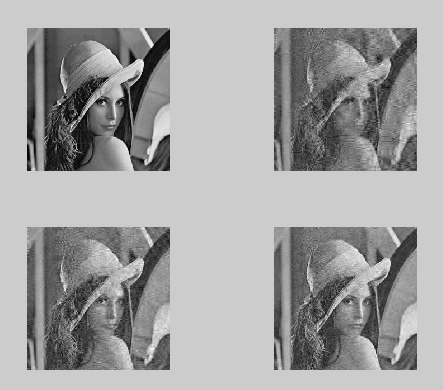psnr和所用时间分别如下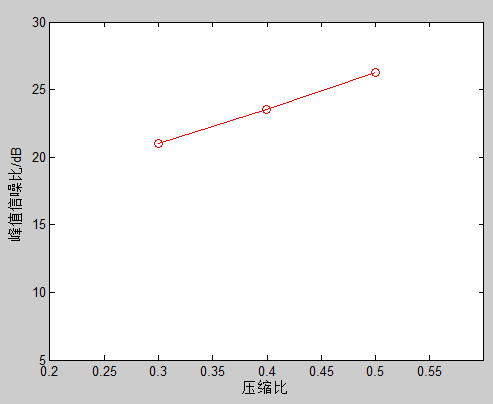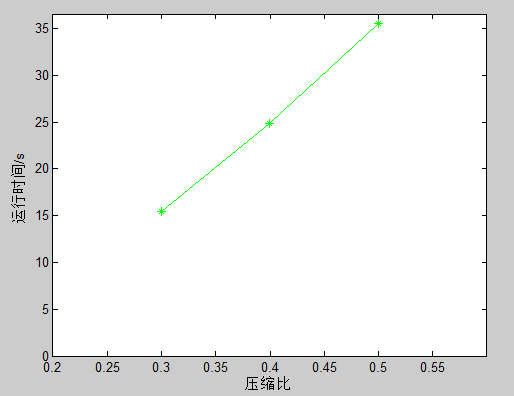书上的图像如下，依次为原图，压缩比为0.5、0.4、0.3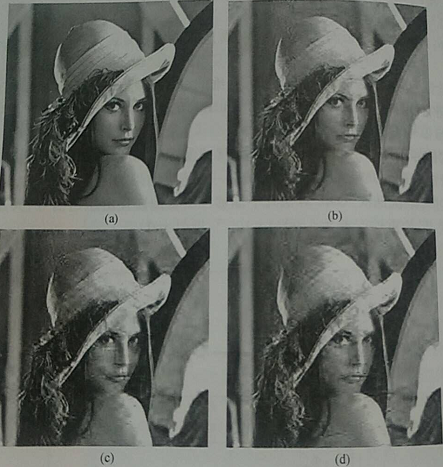所得实际结果感觉较之稍差。

4、结果分析

由于使用的测量矩阵为高斯随机矩阵， 所以每次结果会有偏差，这是部分原因，其余应该还有其它原因。

5、参考文献

1、《压缩感知及应用》，闫敬文 刘蕾 屈小波
2、沙威老师压缩感知代码
3、彬彬有礼的博客，地址如下
http://blog.csdn.net/jbb0523/article/details/45130793

展开全文压缩感知
• 压缩感知算法实现,压缩感知重构算法,matlab源码.zip
• 【实例简介】本工具箱包含常用的压缩感知图像重构算法，如OMP,BP,IHT,等算法，非常齐全。【实例截图】【核心代码】压缩感知图像重构算法├── BCS implemented│ ├── BCSvb.m│ ├── cameraman.pgm│ ├── ...

【实例简介】

本工具箱包含常用的压缩感知图像重构算法，如OMP,BP,IHT,等算法，非常齐全。

【实例截图】

【核心代码】

压缩感知图像重构算法

├── BCS implemented

│   ├── BCSvb.m

│   ├── cameraman.pgm

│   ├── demo_BCSvb_1d.m

│   ├── demo_BCSvb_2d.m

│   ├── demo_BCSvb_2d_small.m

│   └── indor2.pgm

├── compressive sensing basic knowledge

│   ├── anziam_pics.pdf

│   ├── baraniuk-IMA-CScamera-june07.pdf

│   ├── compressed-sensing1.pdf

│   ├── Compressive_Sensing.pdf

│   └── TerryTao.pdf

├── CS_Examples

│   ├── CS_OMP.m

│   ├── CS_SPGL1.m

│   └── spgl1_1_7

│   ├── ChangeLog

│   ├── Contents.m

│   ├── COPYING

│   ├── NormGroupL2_dual.m

│   ├── NormGroupL2_primal.m

│   ├── NormGroupL2_project.m

│   ├── NormL12_dual.m

│   ├── NormL12_primal.m

│   ├── NormL12_project.m

│   ├── NormL1_dual.m

│   ├── NormL1_primal.m

│   ├── NormL1_project.m

│   ├── private

│   │   ├── ensure.m

│   │   ├── heap.c

│   │   ├── heap.h

│   │   ├── lsqr.m

│   │   ├── oneProjectorCore.c

│   │   ├── oneProjectorCore.h

│   │   ├── oneProjector.m

│   │   ├── oneProjectorMex.c

│   │   ├── oneProjectorMex.m

│   │   ├── oneProjectorMex.mexglx

│   │   ├── oneProjectorMex.mexmaci

│   │   └── oneProjectorMex.mexw32

│   ├── spg_bpdn.m

│   ├── spg_bp.m

│   ├── spgdemo.m

│   ├── spg_group.m

│   ├── spgl1.m

│   ├── spg_lasso.m

│   ├── spg_mmv.m

│   ├── spgSetParms.m

│   └── spgsetup.m

├── CS(matlab)

│   └── 分块压缩感知程序(matlab).doc

└── Matlab重构算法

├── CS Recovery Algorithms.pdf

├── software

│   ├── Demo_CS_CoSaMP.m

│   ├── Demo_CS_GBP.m

│   ├── Demo_CS_IHT.m

│   ├── Demo_CS_IRLS.m

│   ├── Demo_CS_OMP.m

│   ├── Demo_CS_SP.m

│   └── lena.bmp

└── SP算法

├── SP.m

└── SP.pdf

9 directories, 58 files

展开全文• 压缩感知重构算法，压缩采样匹配追踪算法matlab仿真代码，供学习交流使用。
• 该代码为压缩感知重构算法的正交匹配追踪的matlab代码，注释很详细OMP 正交匹配追踪
• MATLAB代码如下: 压缩感知仿真实例 压缩感知仿真实例在MATLAB R2001b中的计算结果如下: 原图像 采样率0.7 采样率0.5 采样率0.3 压缩感知仿真实例采用均方误差......MATLAB稀疏表示算法_计算机软件及应用_IT/计算机_...

MATLAB代码如下: 压缩感知仿真实例 压缩感知仿真实例在MATLAB R2001b中的计算结果如下: 原图像 采样率0.7 采样率0.5 采样率0.3 压缩感知仿真实例采用均方误差......

MATLAB稀疏表示算法_计算机软件及应用_IT/计算机_专业资料。关于压缩感知的MATLAB里的信号矩阵稀疏表示算法编码,包含KSVD和OMP算法,比较简单易用 ......

As a companion to these papers, this package includes MATLAB code that implements this recovery procedure in the seven contexts described below. The code ......

该文介绍了压缩感知理论以及 OMP 和 SP 算法的核心思想以及信号的仿真实验, 利用离散小波变换的方法对图像信号进行稀疏化的处理,并分别用 OMP 算法和 SP 算法对......

基于稀疏参数模型及参数先验的图像分辨率 4 结论 通过以上的分析可知 , 在压缩感知理论中 , 用经典基 追踪算法可以有效地在高斯白噪声背景下重构信号 ; 而当 在......

贪婪算法中,SP算法的原理介绍及MATLAB仿真_信息与通信_工程科技_专业资料。贪婪算法中,SP算法的原理介绍及仿真 压缩感知重构算法之子空间追踪(SP) 如果掌握了压缩......

压缩感知的数学理论基础和常用方法,包括稀疏变换、测量矩阵和重构算法,利用Matlab软件实现压缩感知 实验,比较几种测量矩阵的性能,提出双阈值分块正交匹配追踪重构算法。...

基于压缩感知的图像快速... 5页 2下载券 喜欢此文档的还喜欢 混沌信号的压缩感知去噪 8页 免费 基于MATLAB的图像压缩感... 50页 1下载券 基于压缩感知的......

实验表明,该方法具有可操作性和可行性. 关键词 压缩感知;图像去噪;稀疏表示;小波变换;OMP算法 分类号TP39 图像是获取信息和传递信息重要的媒体和 手段,随着计算机......

3 基于小波变换的图像压缩感知本文采用小波变换的方法对信号进行稀疏表示, 利用随机高斯矩阵作为测量 矩阵,最后使用正交匹配法重构信号。Matlab 仿真实验结果如下所示:......

引入 数学实验课程,设计难度适中的实验案例并用 Matlab 语言编程实现.该实验 案例的实施,为学生提供了接触、了解数学前沿研究方法和压缩感知概念的平 台,......

压缩感知理论是编解码思想的一个突 破,减轻了信号采样、传输和存储遇到的巨大压力,是一种信息获取及处理的全 新的理论框架。 本文将利用 MATLAB GUI 进行基于压缩......

《电脑知识与技术》2019 年第 34 期 龙源期刊网 龙源期刊网 摘要:压缩感知即通过信号稀疏性进行全新信号......

基于压缩感知的DOA估计程序_电子/电路_工程科技_专业资料。基于压缩感知的DOA估计程序 程序可运行,有图有真相,MATLAB 得事先装好 cvx 优化包。 clc; clear; ......

《物联网技术》2013 年第 02 期 摘要:为了实现基于压缩感知理论的信号欠采样和重建,采用模拟信息转换器和正交匹配 追踪(Orthogonal Matching Pursuit,OMP)算法对......

稀疏表示是应用压缩感知的先验条件, 随机测量是压缩感知的关键过程,重构算法是获取最终结 果的必要手段。 压缩感知的数学模型 ? 假设x为长度N的一维信号,稀疏度为......

84 传感器与微系统( )TransducerandMicrosystemTechnologies 2018年第37卷第11期 :—()——DOI 10.13873/J.10009787201811008403 基于压缩感知的模拟信息转换器设计*......

压缩感知重构算法之正交匹配追踪(OMP) 前面经过几篇的基础铺垫,本篇给出正交匹配追踪(OMP)算法的 MATLAB 函数代码,并且给出单次测试例程 代码、测量数 M 与重构......

为了改进方向追踪法的重建精度和算法效率, 提出了一种基 于谱投影梯度 (Spectral projected gradient, SPG) 追踪的压缩感知 (Compressed sensing, CS) 重建算法. ......

展开全文• 时域信号压缩传感,正交匹配追踪法（OMP）重构信号,注释比较详细
• 作为1-bit压缩感知重构算法，在此基础上可以仿真，改进稀疏度自适应算法
• % 算法迭代次数(m>=K) Psi=fft(eye(N,N))/sqrt(N); T=Phi*Psi'; hat_y=zeros(1,N); % 待重构的谱域(变换域)向量 Aug_t=[]; % 增量矩阵(初始值为空矩阵) r_n=s; %% 重构 for times=1:m; for col=1:N; ...
• 这是本人编写的基础类的压缩感知重构算法，主要是匹配追踪类算法。
• 压缩感知l1重建算法matlab代码[用于压缩感知恢复的贝叶斯卷积神经网络]。 介绍 贝叶斯卷积神经网络（BCNN）是一种新的压缩感知（CS）恢复算法，它结合了卷积神经网络（CNN）和贝叶斯推理方法。 在本文中，我们显示出...
• 浅谈压缩感知（三十）：压缩感知重构算法之L1最小二乘 主要内容： l1_ls的算法流程l1_ls的MATLAB实现一维信号的实验与结果 前言 前面所介绍的算法都是在匹配追踪算法MP基础上延伸的贪心算法，从本节开始，介绍...
• function Demo_CS_SP() %%%%%%%%%%%%%%%%%%%%%%%%%%%%%%%%%%%%%%%%%%%%%%%%%%%%%%%%%%%%%%%%%%%%%%%%%%% % the DCT basis is selected as the sparse representation dictionary % instead of seting the whole ...
• 附件中是用matlab编写的压缩感知FOCUSS算法，可以运行。FOCUSS算法
• 文档为matlab语言 gpsr算法，亲测有效，文件里有使用说明
• SP(subspace pursuit)算法压缩感知中一种非常重要的贪婪算法，它有较快的计算速度和较好的重构概率，在实际中应用较多。本文给出了SP算法的python和matlab代码，以及完整的仿真过程。参考文献：Dai W, Milenkovic ...
• 算法流程算法分析python代码要利用python实现，电脑必须安装以下程序 python （本文用的python版本为3.5.1） numpy python包（本文用的版本为1.10.4） scipy python包（本文用的版本为0.17.0） pillow python包...python 压缩感知 CoSaMP 重构 matlab
• 本篇介绍压缩感知重构算法GraDes(GradientDescent with Sparsification)，该算法是在文献【GargR, Khandekar R. Gradient descent with sparsification: an iterative algorithmfor sparse recovery with restricted......

压缩感知重构算法matlabmatlab 订阅